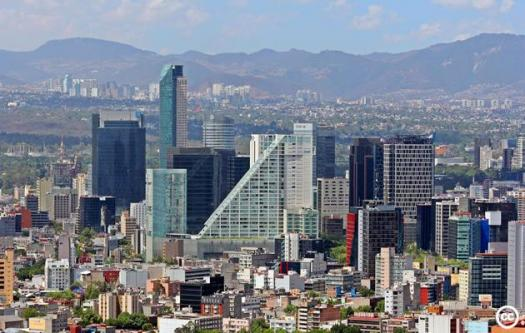# Take The Mexico Quiz

15 Questions | Total Attempts: 81SettingsHow much do you know about our neighbor to the south?

• 1.
Compared to Texas, Mexico is approximately:
• A.

Twice as big

• B.

Three times as big

• C.

Four times as big

• D.

Five times as big

• 2.
Mexico borders:
• A.

The U.S., Honduras and El Salvador

• B.

The U.S., Nicaragua and Costa Rica

• C.

The U.S., Guatemala and El Salvador

• D.

The U.S., Guatemala and Belize

• 3.
Mexico’s highest point is:
• A.

Volcan Pico de Orizaba

• B.

• C.

Volcan Popocatepetl

• D.

Volcan Iztaccihuatl

• 4.
Mexico’s population is roughly:
• A.

30 million

• B.

60 million

• C.

90 million

• D.

120 million

• 5.
Which age group makes up more than 40 percent of the population?
• A.

0-14 years

• B.

15-24 years

• C.

25-54 years

• D.

55-64 years

• 6.
Mexico’s second-largest city by population is:
• A.

Tijuana

• B.

Puebla

• C.

Mexico City

• D.

• 7.
Mexico’s health expenditures, as a percentage of GDP, are approximately:
• A.

3 percent

• B.

6 percent

• C.

9 percent

• D.

12 percent

• 8.
Mexico’s education expenditures, as a percentage of GDP, are approximately:
• A.

5 percent

• B.

10 percent

• C.

20 percent

• D.

25 percent

• 9.
Mexico’s military expenditures, as a percentage of GDP, are approximately:
• A.

0.3 percent

• B.

3 percent

• C.

13 percent

• D.

19 percent

• 10.
Percentage of the population (over 15) who can read and write:
• A.

35 percent

• B.

55 percent

• C.

75 percent

• D.

95 percent

• 11.
How many states does Mexico have?
• A.

11

• B.

21

• C.

31

• D.

41

• 12.
Which of the following is not a Mexican state?
• A.

Campeche

• B.

Colon

• C.

Coahula de Zaragoza

• D.

Colima

• 13.
How many airports does Mexico have?
• A.

17

• B.

171

• C.

571

• D.

1,714

• 14.
How many of Mexico's airports have paved runways?
• A.

7

• B.

11

• C.

243

• D.

571

• 15.
Which of the following is not a Mexican cruise port?
• A.

Altamira

• B.

Cancun

• C.

Cozumel

• D.Back to top